Anúncio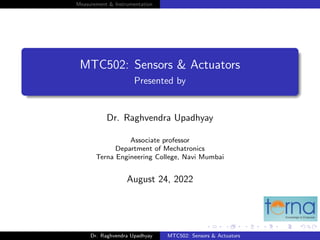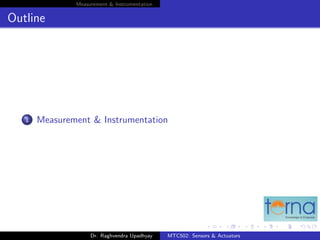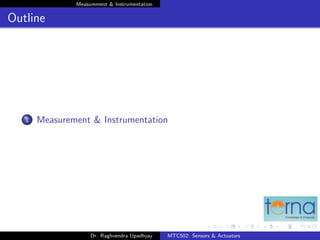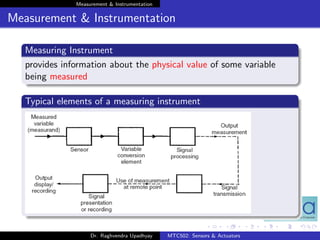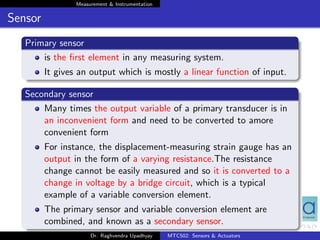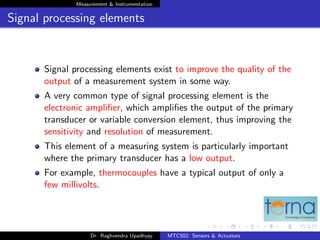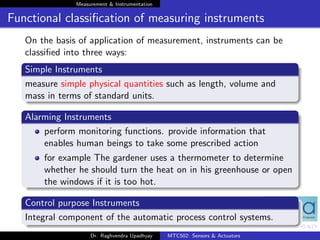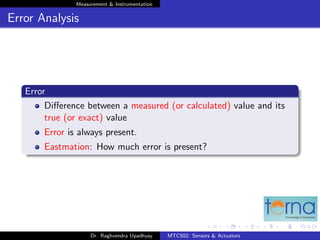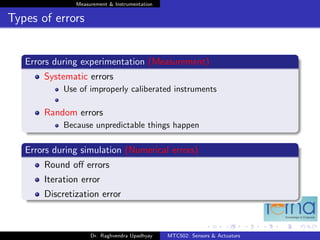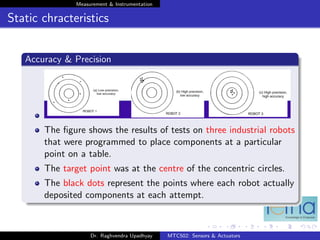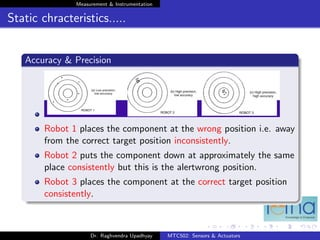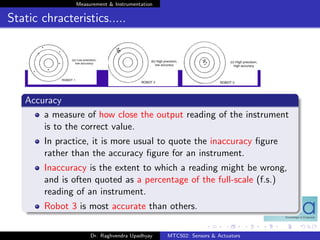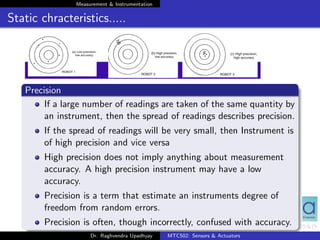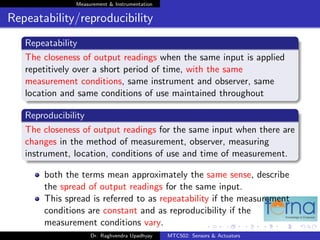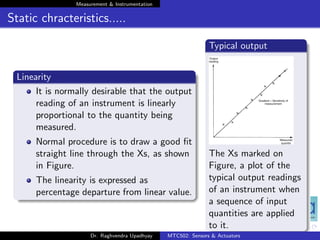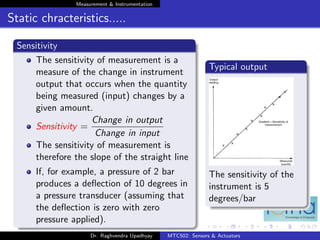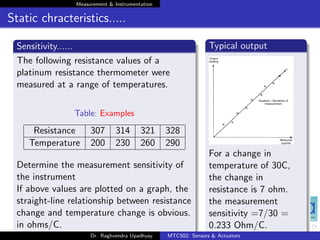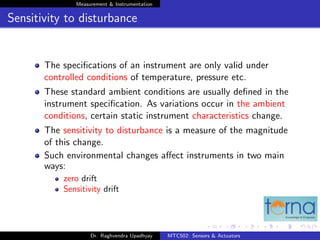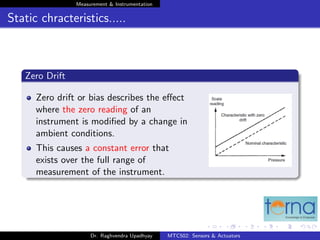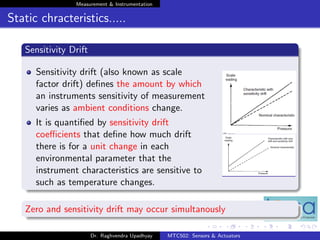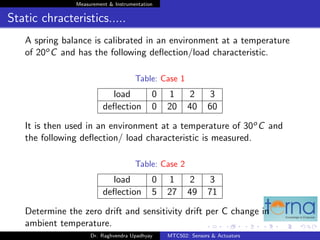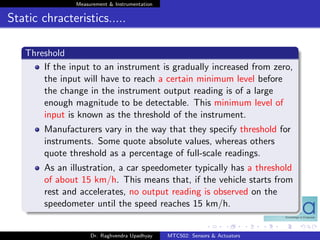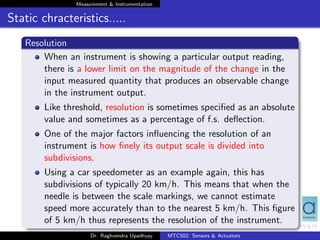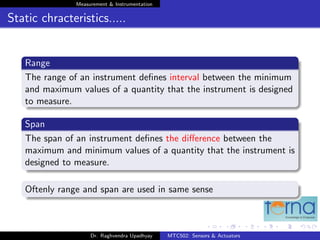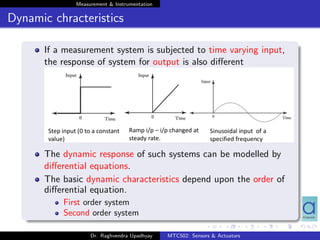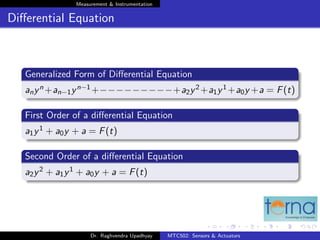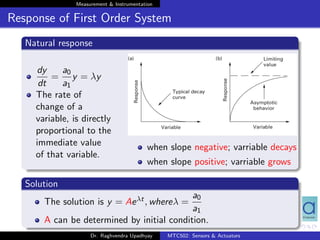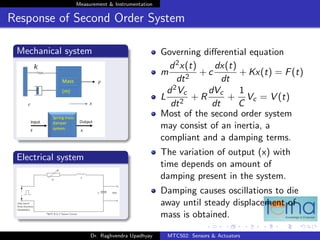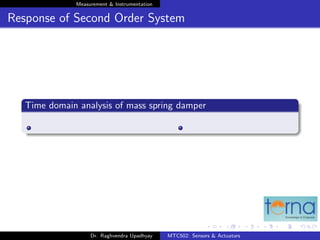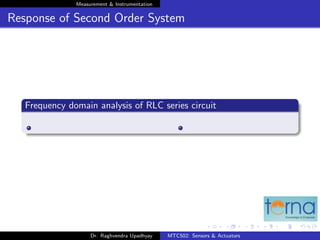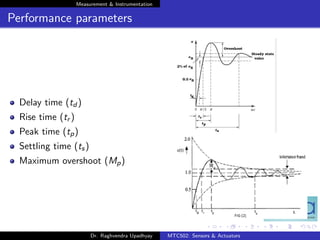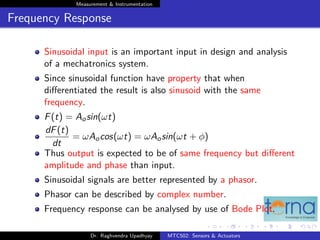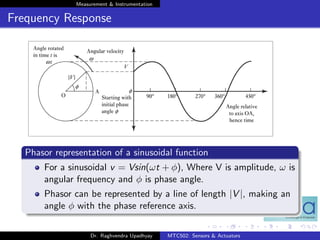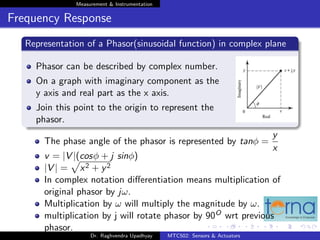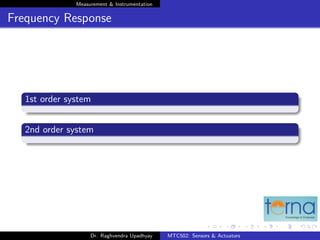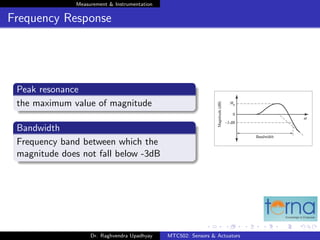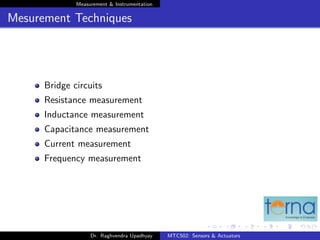1 de 37
Anúncio

### S&A_Characteristics.pdf

1. Measurement & Instrumentation MTC502: Sensors & Actuators Presented by Dr. Raghvendra Upadhyay Associate professor Department of Mechatronics Terna Engineering College, Navi Mumbai August 24, 2022 Dr. Raghvendra Upadhyay MTC502: Sensors & Actuators
2. Measurement & Instrumentation Outline 1 Measurement & Instrumentation Dr. Raghvendra Upadhyay MTC502: Sensors & Actuators
3. Measurement & Instrumentation Outline 1 Measurement & Instrumentation Dr. Raghvendra Upadhyay MTC502: Sensors & Actuators
4. Measurement & Instrumentation Measurement & Instrumentation Measuring Instrument provides information about the physical value of some variable being measured Typical elements of a measuring instrument Dr. Raghvendra Upadhyay MTC502: Sensors & Actuators
5. Measurement & Instrumentation Sensor Primary sensor is the first element in any measuring system. It gives an output which is mostly a linear function of input. Secondary sensor Many times the output variable of a primary transducer is in an inconvenient form and need to be converted to amore convenient form For instance, the displacement-measuring strain gauge has an output in the form of a varying resistance.The resistance change cannot be easily measured and so it is converted to a change in voltage by a bridge circuit, which is a typical example of a variable conversion element. The primary sensor and variable conversion element are combined, and known as a secondary sensor. Dr. Raghvendra Upadhyay MTC502: Sensors & Actuators
6. Measurement & Instrumentation Signal processing elements Signal processing elements exist to improve the quality of the output of a measurement system in some way. A very common type of signal processing element is the electronic amplifier, which amplifies the output of the primary transducer or variable conversion element, thus improving the sensitivity and resolution of measurement. This element of a measuring system is particularly important where the primary transducer has a low output. For example, thermocouples have a typical output of only a few millivolts. Dr. Raghvendra Upadhyay MTC502: Sensors & Actuators
7. Measurement & Instrumentation Functional classification of measuring instruments On the basis of application of measurement, instruments can be classified into three ways: Simple Instruments measure simple physical quantities such as length, volume and mass in terms of standard units. Alarming Instruments perform monitoring functions. provide information that enables human beings to take some prescribed action for example The gardener uses a thermometer to determine whether he should turn the heat on in his greenhouse or open the windows if it is too hot. Control purpose Instruments Integral component of the automatic process control systems. Dr. Raghvendra Upadhyay MTC502: Sensors & Actuators
8. Measurement & Instrumentation Error Analysis Error Difference between a measured (or calculated) value and its true (or exact) value Error is always present. Eastmation: How much error is present? Dr. Raghvendra Upadhyay MTC502: Sensors & Actuators
9. Measurement & Instrumentation Types of errors Errors during experimentation (Measurement) Systematic errors Use of improperly caliberated instruments Random errors Because unpredictable things happen Errors during simulation (Numerical errors) Round off errors Iteration error Discretization error Dr. Raghvendra Upadhyay MTC502: Sensors & Actuators
10. Measurement & Instrumentation Static chracteristics Accuracy & Precision The figure shows the results of tests on three industrial robots that were programmed to place components at a particular point on a table. The target point was at the centre of the concentric circles. The black dots represent the points where each robot actually deposited components at each attempt. Dr. Raghvendra Upadhyay MTC502: Sensors & Actuators
11. Measurement & Instrumentation Static chracteristics..... Accuracy & Precision Robot 1 places the component at the wrong position i.e. away from the correct target position inconsistently. Robot 2 puts the component down at approximately the same place consistently but this is the alertwrong position. Robot 3 places the component at the correct target position consistently. Dr. Raghvendra Upadhyay MTC502: Sensors & Actuators
12. Measurement & Instrumentation Static chracteristics..... Accuracy a measure of how close the output reading of the instrument is to the correct value. In practice, it is more usual to quote the inaccuracy figure rather than the accuracy figure for an instrument. Inaccuracy is the extent to which a reading might be wrong, and is often quoted as a percentage of the full-scale (f.s.) reading of an instrument. Robot 3 is most accurate than others. Dr. Raghvendra Upadhyay MTC502: Sensors & Actuators
13. Measurement & Instrumentation Static chracteristics..... Precision If a large number of readings are taken of the same quantity by an instrument, then the spread of readings describes precision. If the spread of readings will be very small, then Instrument is of high precision and vice versa High precision does not imply anything about measurement accuracy. A high precision instrument may have a low accuracy. Precision is a term that estimate an instruments degree of freedom from random errors. Precision is often, though incorrectly, confused with accuracy. Dr. Raghvendra Upadhyay MTC502: Sensors & Actuators
14. Measurement & Instrumentation Repeatability/reproducibility Repeatability The closeness of output readings when the same input is applied repetitively over a short period of time, with the same measurement conditions, same instrument and observer, same location and same conditions of use maintained throughout Reproducibility The closeness of output readings for the same input when there are changes in the method of measurement, observer, measuring instrument, location, conditions of use and time of measurement. both the terms mean approximately the same sense, describe the spread of output readings for the same input. This spread is referred to as repeatability if the measurement conditions are constant and as reproducibility if the measurement conditions vary. Dr. Raghvendra Upadhyay MTC502: Sensors & Actuators
15. Measurement & Instrumentation Static chracteristics..... Linearity It is normally desirable that the output reading of an instrument is linearly proportional to the quantity being measured. Normal procedure is to draw a good fit straight line through the Xs, as shown in Figure. The linearity is expressed as percentage departure from linear value. Typical output The Xs marked on Figure, a plot of the typical output readings of an instrument when a sequence of input quantities are applied to it. Dr. Raghvendra Upadhyay MTC502: Sensors & Actuators
16. Measurement & Instrumentation Static chracteristics..... Sensitivity The sensitivity of measurement is a measure of the change in instrument output that occurs when the quantity being measured (input) changes by a given amount. Sensitivity = Change in output Change in input The sensitivity of measurement is therefore the slope of the straight line If, for example, a pressure of 2 bar produces a deflection of 10 degrees in a pressure transducer (assuming that the deflection is zero with zero pressure applied). Typical output The sensitivity of the instrument is 5 degrees/bar Dr. Raghvendra Upadhyay MTC502: Sensors & Actuators
17. Measurement & Instrumentation Static chracteristics..... Sensitivity...... The following resistance values of a platinum resistance thermometer were measured at a range of temperatures. Table: Examples Resistance 307 314 321 328 Temperature 200 230 260 290 Determine the measurement sensitivity of the instrument If above values are plotted on a graph, the straight-line relationship between resistance change and temperature change is obvious. in ohms/C. Typical output For a change in temperature of 30C, the change in resistance is 7 ohm. the measurement sensitivity =7/30 = 0.233 Ohm/C. Dr. Raghvendra Upadhyay MTC502: Sensors & Actuators
18. Measurement & Instrumentation Sensitivity to disturbance The specifications of an instrument are only valid under controlled conditions of temperature, pressure etc. These standard ambient conditions are usually defined in the instrument specification. As variations occur in the ambient conditions, certain static instrument characteristics change. The sensitivity to disturbance is a measure of the magnitude of this change. Such environmental changes affect instruments in two main ways: zero drift Sensitivity drift Dr. Raghvendra Upadhyay MTC502: Sensors & Actuators
19. Measurement & Instrumentation Static chracteristics..... Zero Drift Zero drift or bias describes the effect where the zero reading of an instrument is modified by a change in ambient conditions. This causes a constant error that exists over the full range of measurement of the instrument. Dr. Raghvendra Upadhyay MTC502: Sensors & Actuators
20. Measurement & Instrumentation Static chracteristics..... Sensitivity Drift Sensitivity drift (also known as scale factor drift) defines the amount by which an instruments sensitivity of measurement varies as ambient conditions change. It is quantified by sensitivity drift coefficients that define how much drift there is for a unit change in each environmental parameter that the instrument characteristics are sensitive to such as temperature changes. Zero and sensitivity drift may occur simultanously Dr. Raghvendra Upadhyay MTC502: Sensors & Actuators
21. Measurement & Instrumentation Static chracteristics..... A spring balance is calibrated in an environment at a temperature of 20oC and has the following deflection/load characteristic. Table: Case 1 load 0 1 2 3 deflection 0 20 40 60 It is then used in an environment at a temperature of 30oC and the following deflection/ load characteristic is measured. Table: Case 2 load 0 1 2 3 deflection 5 27 49 71 Determine the zero drift and sensitivity drift per C change in ambient temperature. Dr. Raghvendra Upadhyay MTC502: Sensors & Actuators
22. Measurement & Instrumentation Static chracteristics..... Threshold If the input to an instrument is gradually increased from zero, the input will have to reach a certain minimum level before the change in the instrument output reading is of a large enough magnitude to be detectable. This minimum level of input is known as the threshold of the instrument. Manufacturers vary in the way that they specify threshold for instruments. Some quote absolute values, whereas others quote threshold as a percentage of full-scale readings. As an illustration, a car speedometer typically has a threshold of about 15 km/h. This means that, if the vehicle starts from rest and accelerates, no output reading is observed on the speedometer until the speed reaches 15 km/h. Dr. Raghvendra Upadhyay MTC502: Sensors & Actuators
23. Measurement & Instrumentation Static chracteristics..... Resolution When an instrument is showing a particular output reading, there is a lower limit on the magnitude of the change in the input measured quantity that produces an observable change in the instrument output. Like threshold, resolution is sometimes specified as an absolute value and sometimes as a percentage of f.s. deflection. One of the major factors influencing the resolution of an instrument is how finely its output scale is divided into subdivisions. Using a car speedometer as an example again, this has subdivisions of typically 20 km/h. This means that when the needle is between the scale markings, we cannot estimate speed more accurately than to the nearest 5 km/h. This figure of 5 km/h thus represents the resolution of the instrument. Dr. Raghvendra Upadhyay MTC502: Sensors & Actuators
24. Measurement & Instrumentation Static chracteristics..... Range The range of an instrument defines interval between the minimum and maximum values of a quantity that the instrument is designed to measure. Span The span of an instrument defines the difference between the maximum and minimum values of a quantity that the instrument is designed to measure. Oftenly range and span are used in same sense Dr. Raghvendra Upadhyay MTC502: Sensors & Actuators
25. Measurement & Instrumentation Dynamic chracteristics If a measurement system is subjected to time varying input, the response of system for output is also different The dynamic response of such systems can be modelled by differential equations. The basic dynamic characteristics depend upon the order of differential equation. First order system Second order system Dr. Raghvendra Upadhyay MTC502: Sensors & Actuators
26. Measurement & Instrumentation Differential Equation Generalized Form of Differential Equation anyn +an−1yn−1 +−−−−−−−−−+a2y2 +a1y1 +a0y +a = F(t) First Order of a differential Equation a1y1 + a0y + a = F(t) Second Order of a differential Equation a2y2 + a1y1 + a0y + a = F(t) Dr. Raghvendra Upadhyay MTC502: Sensors & Actuators
27. Measurement & Instrumentation Response of First Order System Natural response dy dt = a0 a1 y = λy The rate of change of a variable, is directly proportional to the immediate value of that variable. when slope negative; varriable decays when slope positive; varriable grows Solution The solution is y = Aeλt, whereλ = a0 a1 A can be determined by initial condition. Dr. Raghvendra Upadhyay MTC502: Sensors & Actuators
28. Measurement & Instrumentation Response of Second Order System Mechanical system Electrical system Governing differential equation m d2x(t) dt2 + c dx(t) dt + Kx(t) = F(t) L d2Vc dt2 + R dVc dt + 1 C Vc = V (t) Most of the second order system may consist of an inertia, a compliant and a damping terms. The variation of output (x) with time depends on amount of damping present in the system. Damping causes oscillations to die away until steady displacement of mass is obtained. Dr. Raghvendra Upadhyay MTC502: Sensors & Actuators
29. Measurement & Instrumentation Response of Second Order System Time domain analysis of mass spring damper Dr. Raghvendra Upadhyay MTC502: Sensors & Actuators
30. Measurement & Instrumentation Response of Second Order System Frequency domain analysis of RLC series circuit Dr. Raghvendra Upadhyay MTC502: Sensors & Actuators
31. Measurement & Instrumentation Performance parameters Delay time (td ) Rise time (tr ) Peak time (tp) Settling time (ts) Maximum overshoot (Mp) Dr. Raghvendra Upadhyay MTC502: Sensors & Actuators
32. Measurement & Instrumentation Frequency Response Sinusoidal input is an important input in design and analysis of a mechatronics system. Since sinusoidal function have property that when differentiated the result is also sinusoid with the same frequency. F(t) = Aosin(ωt) dF(t) dt = ωAocos(ωt) = ωAosin(ωt + φ) Thus output is expected to be of same frequency but different amplitude and phase than input. Sinusoidal signals are better represented by a phasor. Phasor can be described by complex number. Frequency response can be analysed by use of Bode Plot. Dr. Raghvendra Upadhyay MTC502: Sensors & Actuators
33. Measurement & Instrumentation Frequency Response Phasor representation of a sinusoidal function For a sinusoidal v = Vsin(ωt + φ), Where V is amplitude, ω is angular frequency and φ is phase angle. Phasor can be represented by a line of length |V |, making an angle φ with the phase reference axis. Dr. Raghvendra Upadhyay MTC502: Sensors & Actuators
34. Measurement & Instrumentation Frequency Response Representation of a Phasor(sinusoidal function) in complex plane Phasor can be described by complex number. On a graph with imaginary component as the y axis and real part as the x axis. Join this point to the origin to represent the phasor. The phase angle of the phasor is represented by tanφ = y x v = |V |(cosφ + j sinφ) |V | = p x2 + y2 In complex notation differentiation means multiplication of original phasor by jω. Multiplication by ω will multiply the magnitude by ω. multiplication by j will rotate phasor by 90O wrt previous phasor. Dr. Raghvendra Upadhyay MTC502: Sensors & Actuators
35. Measurement & Instrumentation Frequency Response 1st order system 2nd order system Dr. Raghvendra Upadhyay MTC502: Sensors & Actuators
36. Measurement & Instrumentation Frequency Response Peak resonance the maximum value of magnitude Bandwidth Frequency band between which the magnitude does not fall below -3dB Dr. Raghvendra Upadhyay MTC502: Sensors & Actuators
37. Measurement & Instrumentation Mesurement Techniques Bridge circuits Resistance measurement Inductance measurement Capacitance measurement Current measurement Frequency measurement Dr. Raghvendra Upadhyay MTC502: Sensors & Actuators
Anúncio